Function Repository Resource:

# LatticeVoronoiCellPlot3D

Display Voronoi cells for specific lattice points in a 3D lattice

 ResourceFunction["LatticeVoronoiCellPlot3D"][{vx,vy,vz},{nx,ny,nz}] plots the Voronoi cells around each lattice point for the lattice defined by the basis vectors vi and with the number of cells in each direction specified by the ni.

## Details and Options

Each vi is a vector in three dimensions.
The ni are natural numbers that specify the number of lattice points to be drawn along each of the corresponding basis vector directions.
ResourceFunction["LatticeVoronoiCellPlot3D"] takes the option "UnitCellsToShow", which is a list of indices specifying which Voronoi cells to plot (highlight). The default is to highlight all of the unit cells.

## Examples

### Basic Examples (5)

Plot the Voronoi cells for a given hexagonal lattice:

 In:=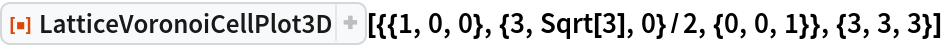Out=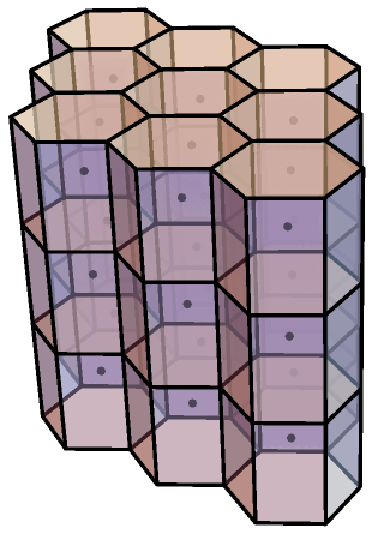Plot the Voronoi cells for a cubic lattice:

 In:=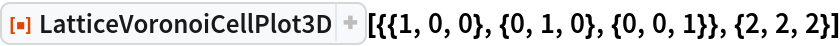Out=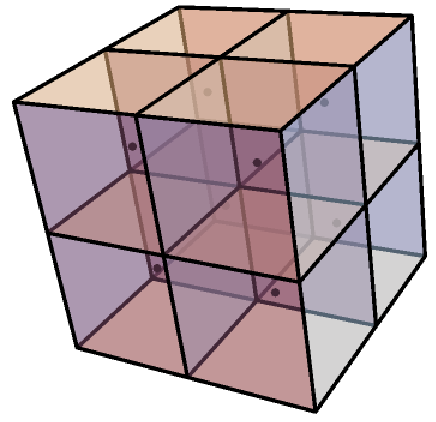Plot the Voronoi cells for a body-centred cubic lattice:

 In:=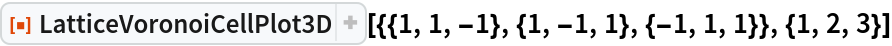Out=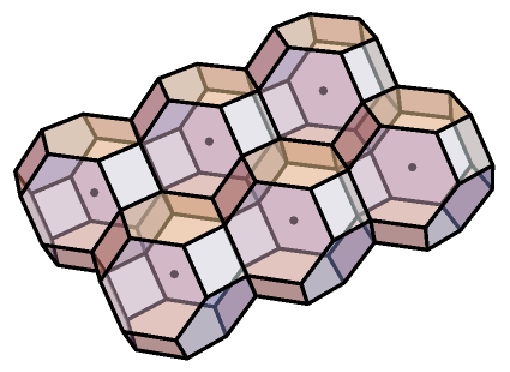Plot the Voronoi cells for a body-centred tetragonal lattice:

 In:=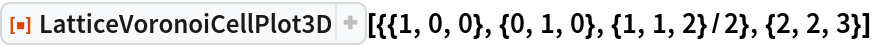Out=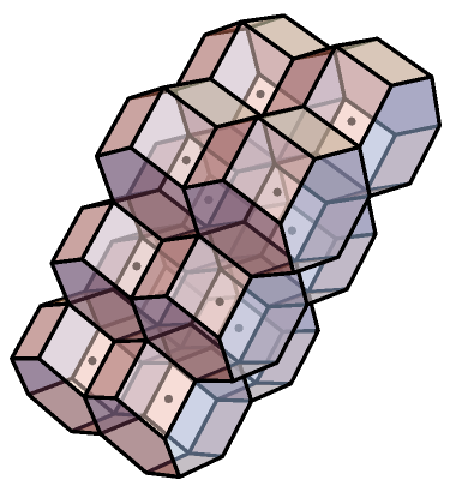Plot the Voronoi cells for a face-centred cubic lattice:

 In:=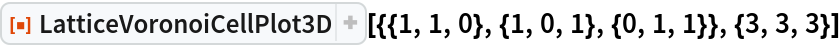Out=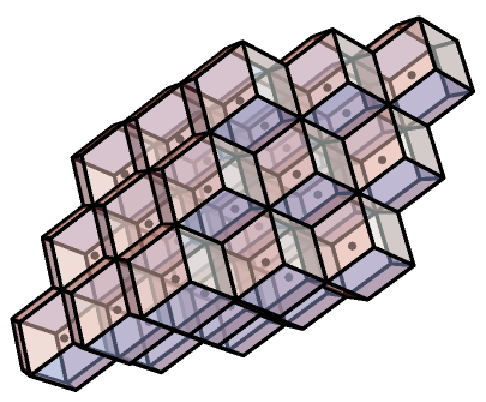### Options (1)

#### UnitCellsToShow (1)

Specify the indices of the Voronoi cells to be highlighted by using the option "UnitCellsToShow":

 In:=Out=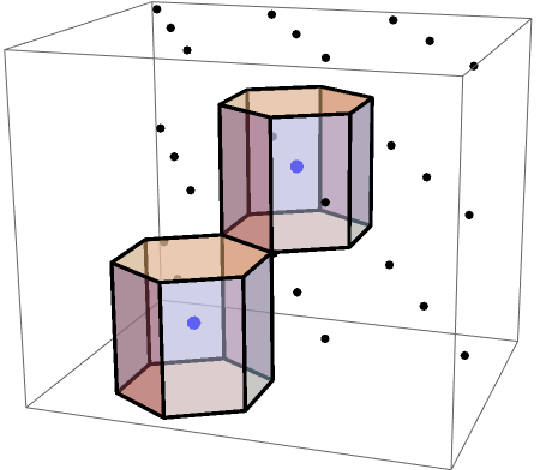## Publisher

WolframSpecialProjects

## Version History

• 1.0.0 – 04 November 2020